C### Details

Languages
English
Kindergarten, Pre-K, 1st
Resource Type
Activities, Math Centers, Printables
Product
Digital
Pages
35 Pages
Subject
Basic Math, Counting
Standard
Common Core Mathematics Standards

### Description

Are you looking for an engaging set of no prep worksheets and centers that focus on a specific number? This is full of mazes, cut and paste printables, cutting numbers, dot to dot of the number, puzzles, and color by code number word and numeral pages. Dive into the number 0 with this ocean themed 35 page printable pdf full of number sense worksheets and activities.

This has 19 printables that include worksheets, mazes, dot to dots, color by number sense, number sense puzzles and a cutting practice center. The puzzles come in color and BW.

Students will practice modeling the number with ten frames, identifying the number on a 120 chart, trace the number word, trace the numeral, counting order of one more and one less, number and number word color by codes using different fonts, cut and paste to spell the number word, follow a counting order to complete a dot to dot, subitizing dots, tally marks, cutting practice, and find the number on a number line.

✨MY PRODUCT INCLUDES✨
7 Worksheets
3 Cut and Paste Worksheets
4 Color by Codes for Number Name and Numeral
4 Puzzles 2 in Color and 2 in BW
1 Cutting Out the Number

WHAT IS INCLUDED ON THE WORKSHEETS?
trace the counters
trace the number
color the correct amount of jellyfish
find the number on a number line
color the correct number of fish
trace the number
trace the number word
find the number in a wordsearch
model the number on a ten frame
complete the number dot to dot
find the number on a 120 chart
follow the number maze in an octopus shape
harder number shapes maze
number color by code using different fonts (including ten frames, hands, and tally marks)
number word color by code using different fonts (including ten frames, hands, and tally marks)
spell the number word
identify the numbers
find the hidden numbers
one more than the number
one less than the number

WHAT IS INCLUDED ON THE CUT AND PASTE WORKSHEETS?
counting items
coloring the correct amount of items
spelling the number word
1 more and 1 less
subitizing dots
tally marks

WHAT IS INCLUDED WITH THE CENTERS?
cut and paste puzzle in color and BW that includes the number, number word, and tracing the number and number word.
cutting out number practice that includes the number, number word, and tracing the number and number word.

THIS PRODUCT FOCUSES ON THE FOLLOWING STANDARDS:
✅Common Core State Standards
K.CC.A.2 Count forward beginning from a given number within the known sequence (instead of having to begin at 1).
K.CC.A.3 Write numbers from 0 to 20. Represent a number of objects with a written numeral 0-20 (with 0 representing a count of no objects).
K.CC.B.4 Understand the relationship between numbers and quantities; connect counting to cardinality.
K.CC.B.4.A When counting objects, say the number names in the standard order, pairing each object with one and only one number name and each number name with one and only one object.
K.CC.B.4.B Understand that the last number name said tells the number of objects counted. The number of objects is the same regardless of their arrangement or the order in which they were counted.
K.CC.B.5 Count to answer “how many?” questions about as many as 20 things arranged in a line, a rectangular array, or a circle, or as many as 10 things in a scattered configuration; given a number from 1-20, count out that many objects.

✅Texas Essential Knowledge and Skills
MA.K.2.A Number and operations. The student applies mathematical process standards to understand how to represent and compare whole numbers, the relative position and magnitude of whole numbers, and relationships within the numeration system. The student is expected to: count forward and backward to at least 20 with and without objects;
MA.K.2.B Number and operations. The student applies mathematical process standards to understand how to represent and compare whole numbers, the relative position and magnitude of whole numbers, and relationships within the numeration system. The student is expected to: read, write, and represent whole numbers from 0 to at least 20 with and without objects or pictures;
MA.K.2.C Number and operations. The student applies mathematical process standards to understand how to represent and compare whole numbers, the relative position and magnitude of whole numbers, and relationships within the numeration system. The student is expected to: count a set of objects up to at least 20 and demonstrate that the last number said tells the number of objects in the set regardless of their arrangement or order;
MA.K.2.D Number and operations. The student applies mathematical process standards to understand how to represent and compare whole numbers, the relative position and magnitude of whole numbers, and relationships within the numeration system. The student is expected to: recognize instantly the quantity of a small group of objects in organized and random arrangements;
MA.K.2.F Number and operations. The student applies mathematical process standards to understand how to represent and compare whole numbers, the relative position and magnitude of whole numbers, and relationships within the numeration system. The student is expected to: generate a number that is one more than or one less than another number up to at least 20;

✅Virginia Standards of Learning
NS.K.1.a The student will tell how many are in a given set of 20 or fewer objects by counting orally; and
NS.K.1.b The student will read, write, and represent numbers from 0 through 20.

QUESTIONS OR CONNECT WITH ME
✉ EMAIL [email protected]
✍MESSAGE ME:
www.dragonfly-bay.com
www.dragonflybaylearning.com

❤️Thank you, Michelle-Dragonfly Bay
Are you looking for an engaging set of no prep worksheets and centers that focus on a specific number? This is full of mazes, cut and paste printables, cutting numbers, dot to dot of the number, puzzles, and color by... more

### Reviews

0
Overall review score

Displaying All Reviews | 0 Reviews

0
0%
0
0%
0
0%
0
0%
0
0%

### More from this shop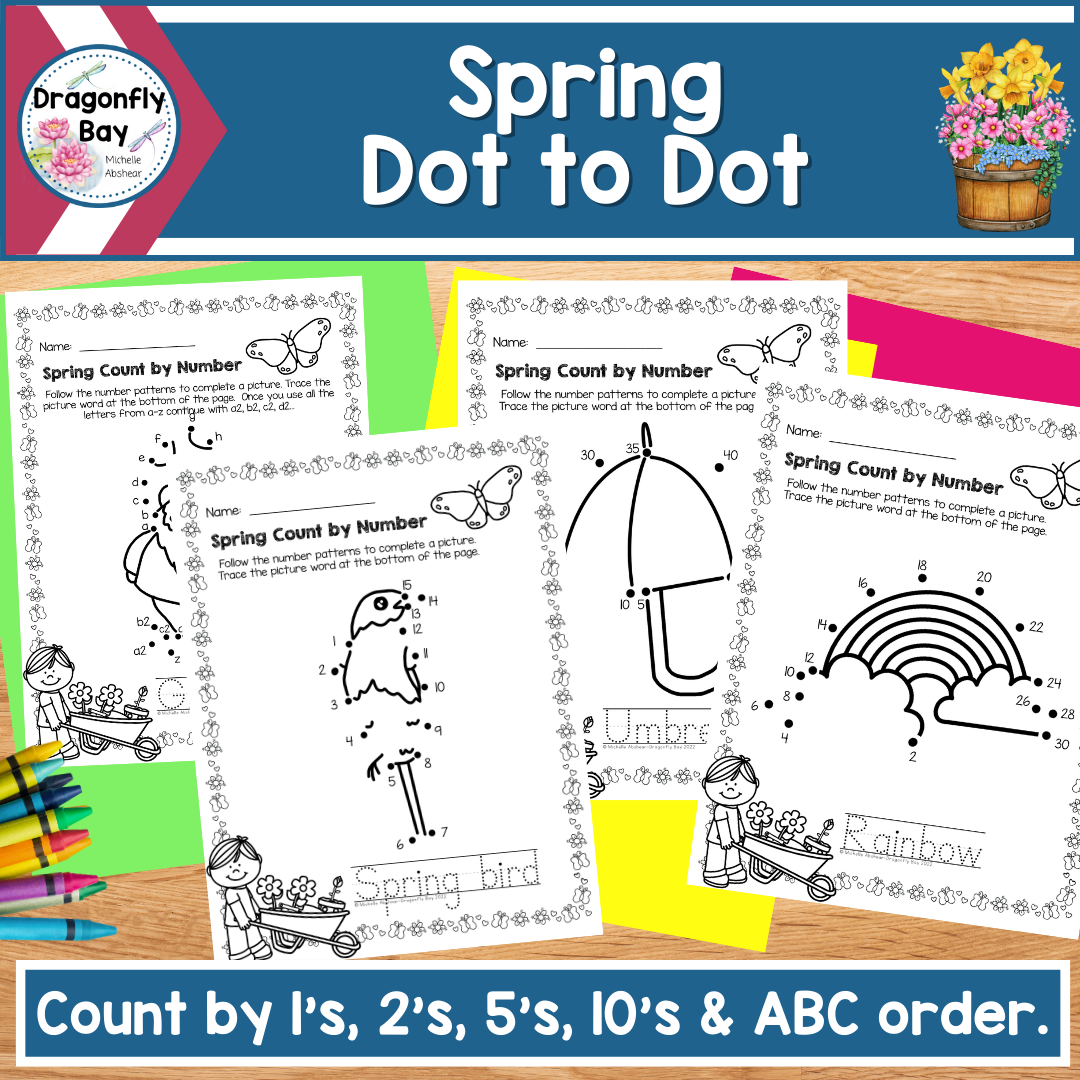\$6.00\$4.00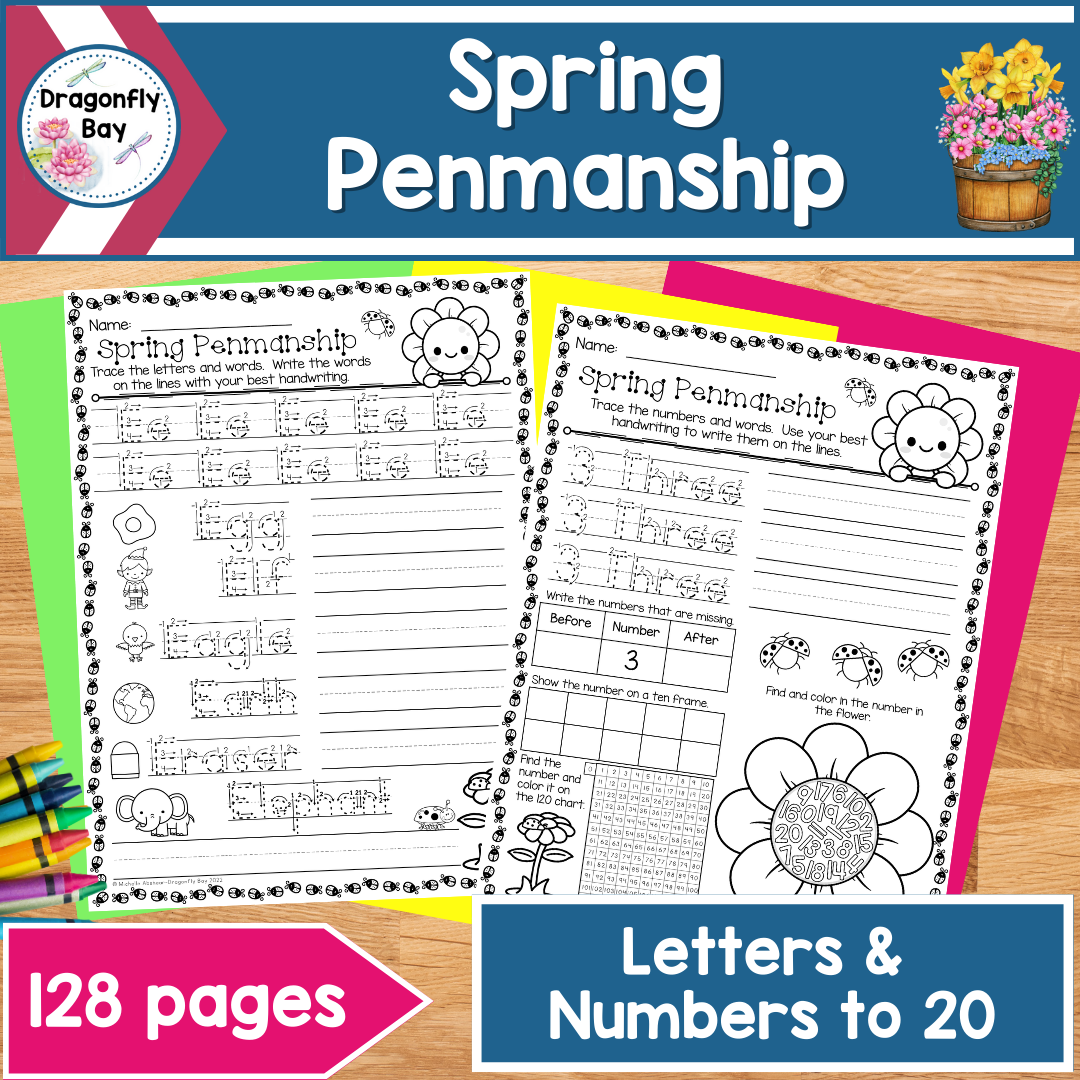\$6.00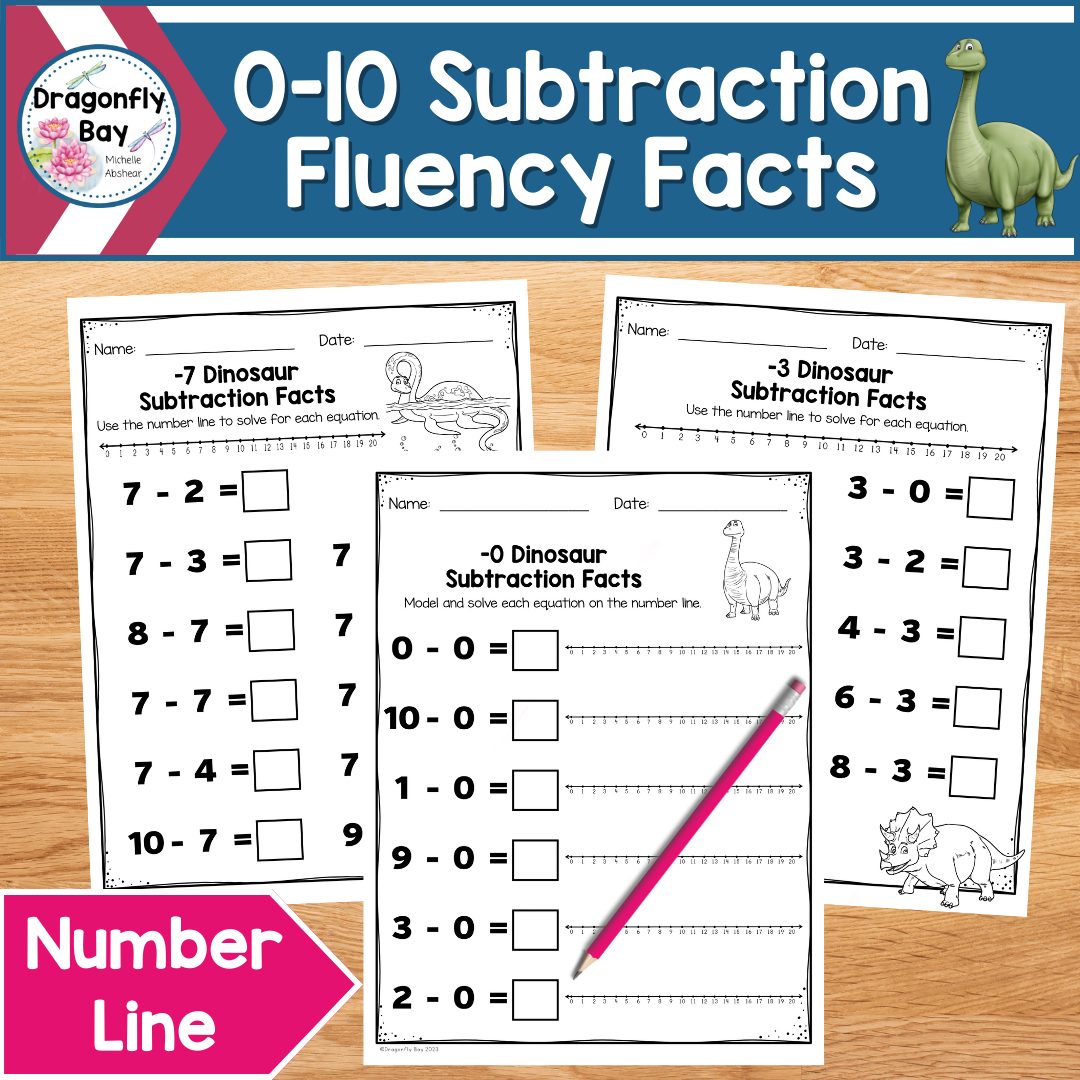\$4.00
\$5.00
-20% Off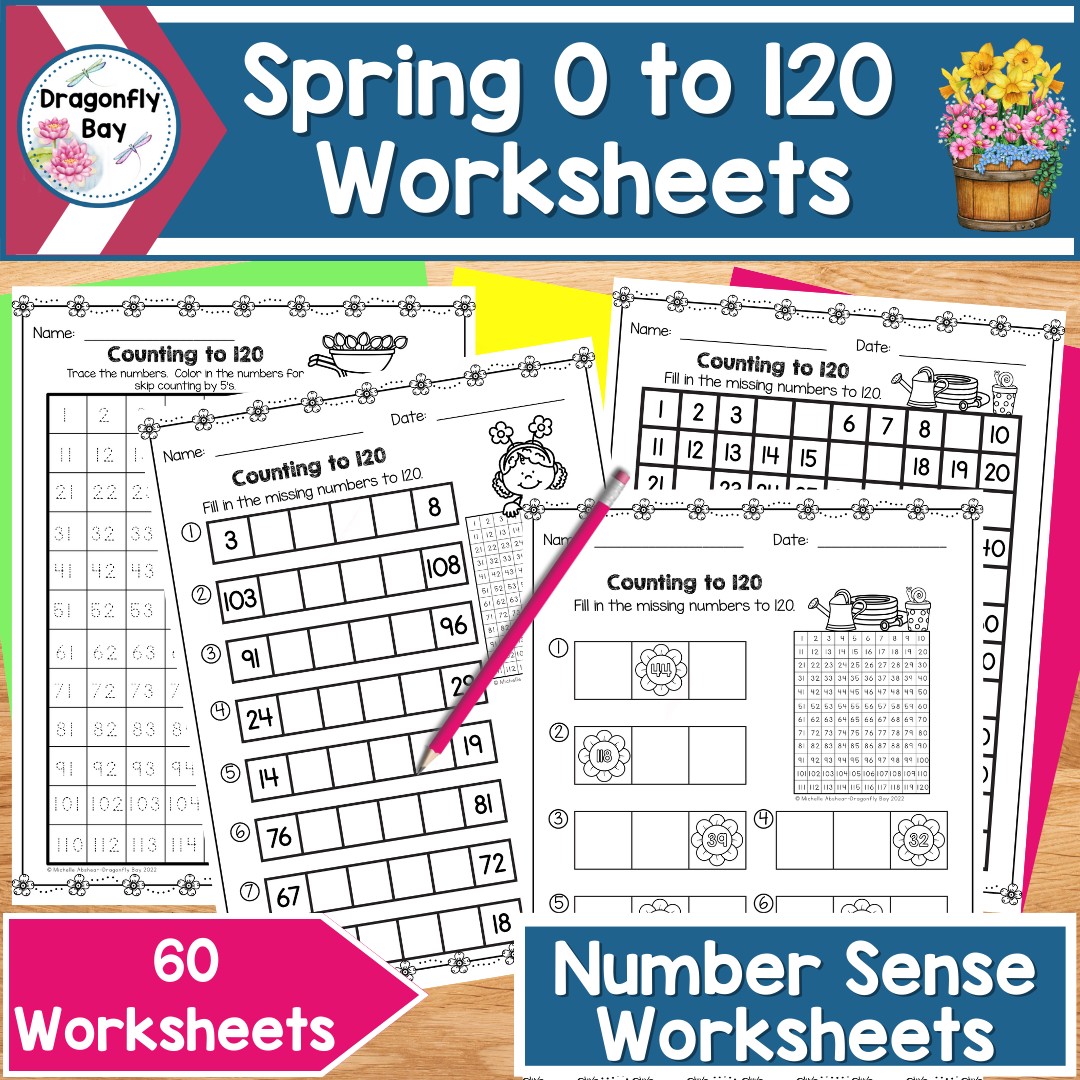\$5.00\$6.00\$4.00\$2.00\$4.50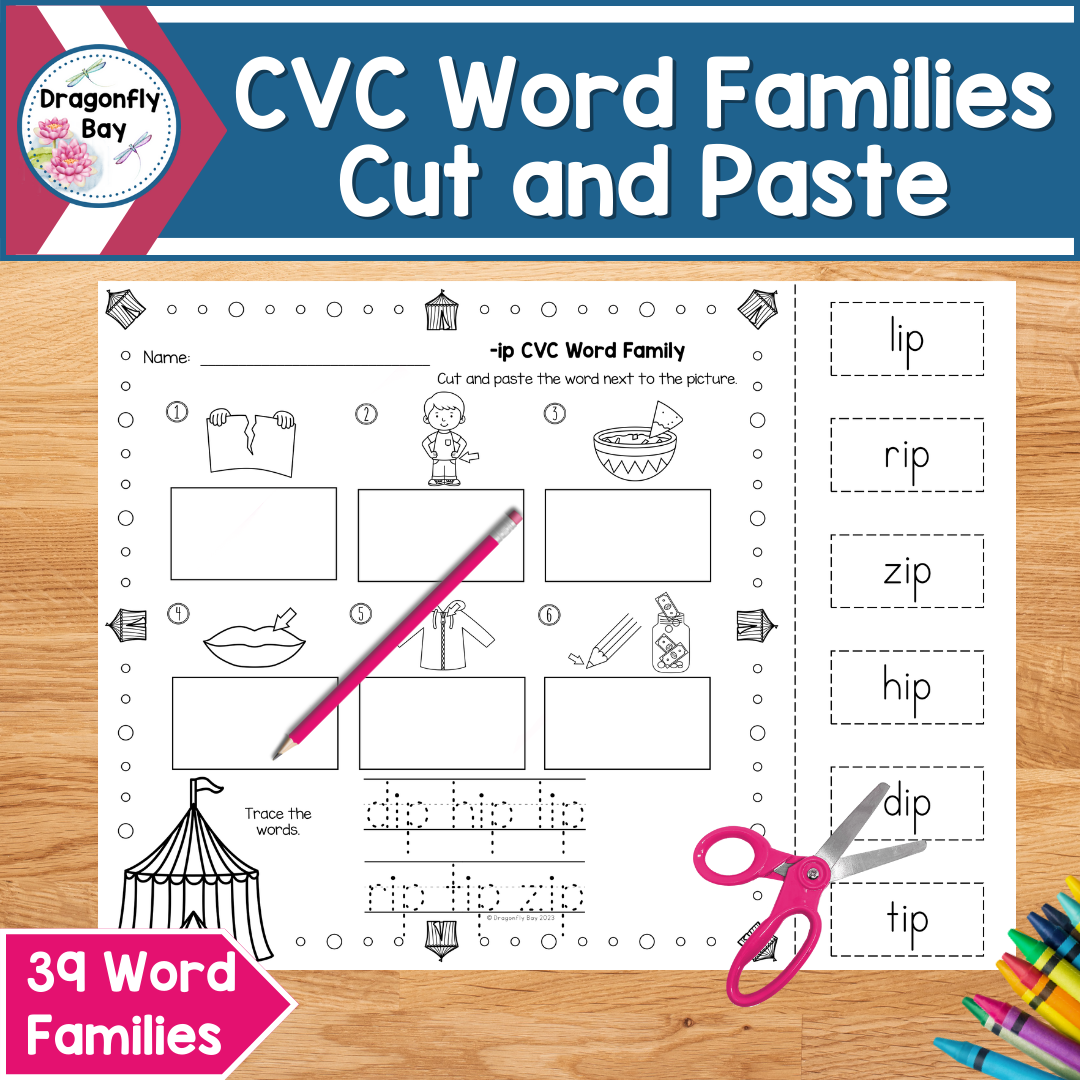\$3.00\$2.00\$4.00\$4.00\$6.00\$6.00

Shop | Product

Dragonfly Bay
\$0.00